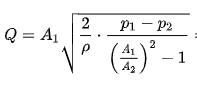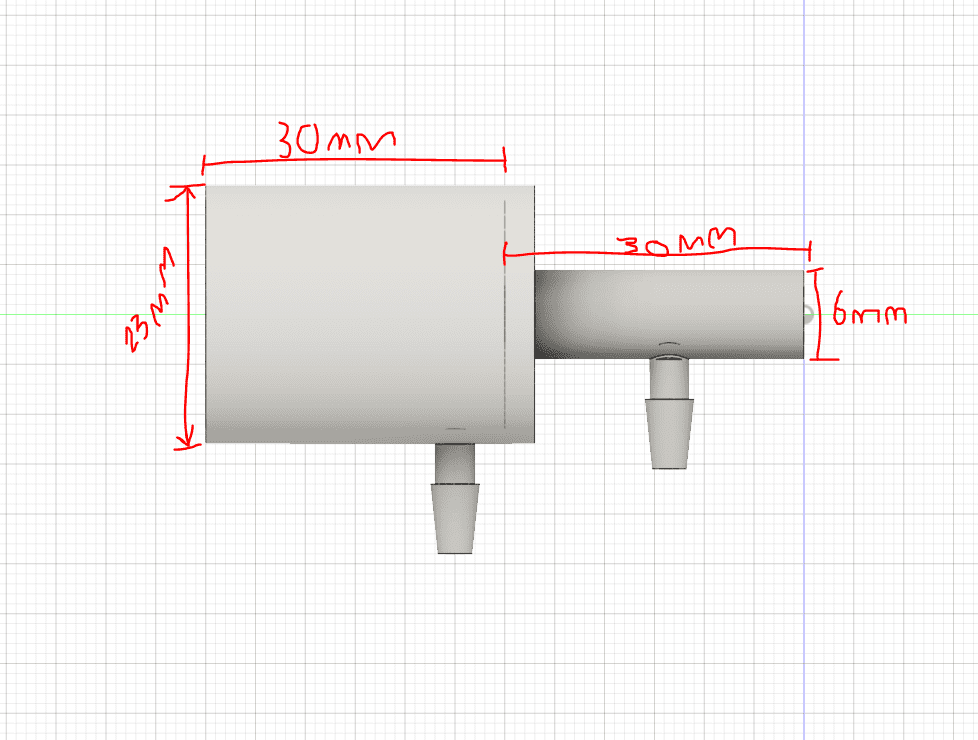# Volumetric Flow Rate through a Venturi Meter

• Teeg

#### Teeg

TL;DR Summary
TL DR: Am I using correct formula for calculating Volumetric Flow rate through Venturi Tube
Hi, I have designed a simple Venturi meter to measure pressure differential across to section of the pipe as pictured. However in calculations I am not sure if I am doing something wrong. I am trying to calculate Volumetric flow rate in L/s.I am using Bernoulli Equation of the Venturi meter as above

From my MPX5010 DP Sensor I am getting a pressure differential of 222.2 pa.

A1 is cross sectional area of first part of the tube = Pi * R^2 = 0.000415 M^2

A2 = Pi* R^2 = 0.0000283 M^2

P = Density of air = 1.225

P1-P2 = DeltaP = 222.2 Pa

Plugging all into the equation above I am getting a volumetric flowrate = 0.000539783 M^3/s

Multiply by 1000 = 0.539783 L/s

Is this correct, is the formula correct ? I have seen multiple different formulas stipulated online.

Thank youFormula is as it is shown here . What others did you find ?
When I do the calculation I get your result -- but with less digits.

•Teeg and Lnewqban
Thanks for your response. Looking further into the topic I think I am not achieving laminar flow and this is my problem, I am going to re design the tube to attempt to achieve laminar flow through the device.

## What is a Venturi meter and how does it work?

A Venturi meter is a type of flow meter used to measure the volumetric flow rate of a fluid. It consists of a constricted section in a pipe, known as a Venturi, which causes a pressure drop as fluid flows through it. This pressure drop is used to calculate the flow rate using Bernoulli's principle.

## What factors affect the accuracy of a Venturi meter?

The accuracy of a Venturi meter can be affected by the fluid density, fluid viscosity, and the shape and dimensions of the meter. Additionally, any obstructions or bends in the pipe leading to the meter can also impact its accuracy.

## How do you calculate the volumetric flow rate using a Venturi meter?

The volumetric flow rate through a Venturi meter can be calculated using the following formula: Q = A1*V1 = A2*V2, where Q is the flow rate, A1 and A2 are the cross-sectional areas of the pipe before and after the Venturi, and V1 and V2 are the fluid velocities before and after the Venturi, respectively.

## What are the advantages of using a Venturi meter?

Venturi meters have several advantages, including high accuracy, minimal pressure loss, and low maintenance requirements. They are also suitable for measuring a wide range of flow rates and can handle fluids with high viscosity or containing suspended solids.

## What are some common applications of Venturi meters?

Venturi meters are commonly used in various industries, including oil and gas, water treatment, chemical processing, and HVAC systems. They are also used in laboratory settings for measuring the flow rate of gases and liquids in experiments.